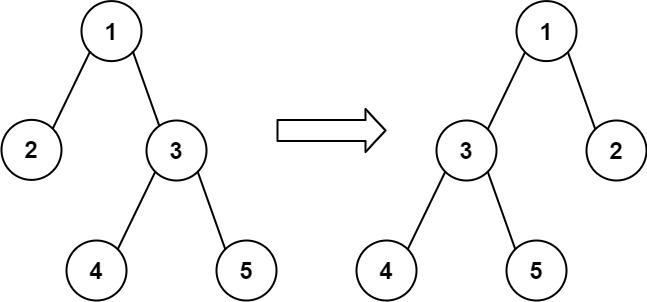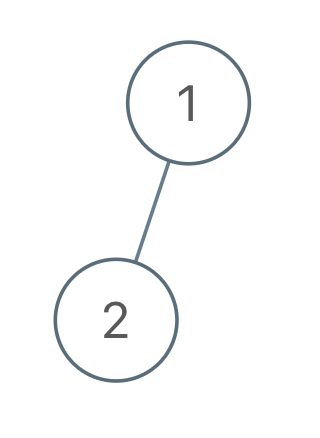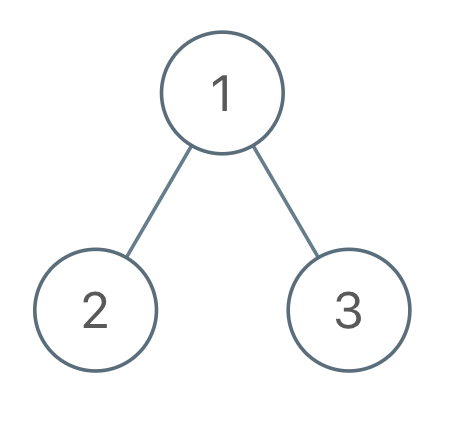## Algorithm

Problem Name: 971. Flip Binary Tree To Match Preorder Traversal

You are given the `root` of a binary tree with `n` nodes, where each node is uniquely assigned a value from `1` to `n`. You are also given a sequence of `n` values `voyage`, which is the desired pre-order traversal of the binary tree.

Any node in the binary tree can be flipped by swapping its left and right subtrees. For example, flipping node 1 will have the following effect:Flip the smallest number of nodes so that the pre-order traversal of the tree matches `voyage`.

Return a list of the values of all flipped nodes. You may return the answer in any order. If it is impossible to flip the nodes in the tree to make the pre-order traversal match `voyage`, return the list `[-1]`.

Example 1:```Input: root = [1,2], voyage = [2,1]
Output: [-1]
Explanation: It is impossible to flip the nodes such that the pre-order traversal matches voyage.
```

Example 2:```Input: root = [1,2,3], voyage = [1,3,2]
Output: 
Explanation: Flipping node 1 swaps nodes 2 and 3, so the pre-order traversal matches voyage.```

Example 3:```Input: root = [1,2,3], voyage = [1,2,3]
Output: []
Explanation: The tree's pre-order traversal already matches voyage, so no nodes need to be flipped.
```

Constraints:

• The number of nodes in the tree is `n`.
• `n == voyage.length`
• `1 <= n <= 100`
• `1 <= Node.val, voyage[i] <= n`
• All the values in the tree are unique.
• All the values in `voyage` are unique.

## Code Examples

### #1 Code Example with Javascript Programming

```Code - Javascript Programming```

``````
const flipMatchVoyage = function (root, voyage) {
const n = voyage.length
const res = []
let idx = 0
return dfs(root, 0) ? res : [-1]

function dfs(node) {
if (node == null) return true
if (node.val !== voyage[idx]) {
return false
}
idx++
if (node.left && node.left.val !== voyage[idx]) {
res.push(node.val)
return dfs(node.right) && dfs(node.left)
}
return dfs(node.left) && dfs(node.right)
}
}
``````
Copy The Code &

Input

cmd
root = [1,2,3], voyage = [1,3,2]

Output

cmd


### #2 Code Example with Python Programming

```Code - Python Programming```

``````
class Solution:
def flipMatchVoyage(self, root, voyage):
res = []
self.i = 0
def dfs(node):
if not node: return True
if node.val != voyage[self.i]:
return False
self.i += 1
if node.left and node.left.val != voyage[self.i]:
node.left, node.right = node.right, node.left
res.append(node.val)
return dfs(node.left) and dfs(node.right)
return res if dfs(root) else [-1]
``````
Copy The Code &

Input

cmd
root = [1,2,3], voyage = [1,3,2]

Output

cmd